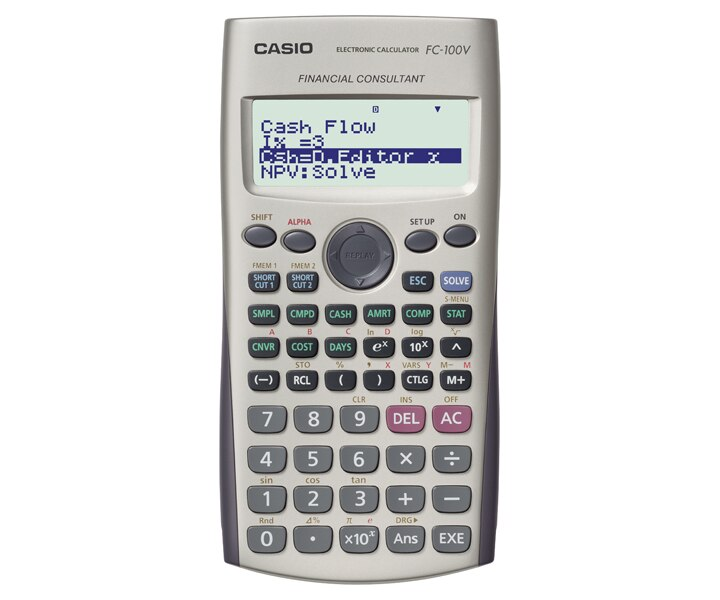•Casio Calculator (FC200V)

Regular price
Rs 12,950.00 LKR
Regular price
Sale price
Rs 12,950.00 LKR

•10 + 2 digits10-digit mantissa + 2-digit exponential display.

•Solar & BatterySolar powered when light is sufficient, battery powered when light is insufficient.

•Key rolloverKey operations are stored in a buffer, so nothing is lost even during high-speed input.

•--Simple interest

• Interest amount, principal and interest

Compound interest

• Payment period, interest rate, deposit amount, future value

Investment Appraisal (cash flow)

• Net present value method, internal rate of return method, payback period method, etc.

Amortization

• Monthly payment, principal and interest to date

General and function

• Virtually the same functions as a standard calculator.

Statistical and regression

• Statistical calculations using input sample data.

Interest rate conversion

• Nominal interest rate and effective interest rate conversion

Cost, selling price,or margin

• Calculation of any of the above values after inputting the other two

Day or date calculations

• Virtually the same as a standard calculator, with some variation in the input method

Depreciation

• Straight-line method, declining balance method

Bond calculation

• Purchase price, annual yield

Break-even point

• Six modes for calculation of break-even point, etc